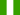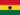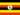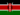This Course has been revised!

For a more enjoyable learning experience, we recommend that you study the mobile-friendly republished version of this course.

Take me to revised course.

- or -

Continue studying this course

Negative correlation

• Study Notes
• Discuss This TopicLAWRENCE M. 0 0 In contrast to a positive correlation, a negative correlation indicates an inverse relationship between two variables.Peter Awuni A. 0 0 What is the difference between the negative and scatter correlationPeter Awuni A. 0 0 What is the difference between negative and scatter correlation?Peter Awuni A. 0 0 What is the significance of negative correlation?Peter Awuni A. 0 0 Which of the following correlation is best for researchers to draw accurate assumptions?Tamekia Dominquie T. 0 0 Negative correlation example, there is not enough in the gameOdongo M. 0 0 Psychology -> Negative correlation Negative correlation In contrast to a positive correlation, a negative correlation indicates an inverse relationship between two variables. This means that a high score on variable X is associated with a low score on variable Y, or a low score on variable X is associated with a high score on variable Y. For example, one could predict a negative correlation between the number of police patrolling the streets and the rate of crime in that area. The dots on the scatter diagram would still cluster together but would indicate that as one variable increases in value the other variable decreases in value. The correlation method is useful to a researcher as it enables the researcher to make predictions. It indicates if a relationship exists between two variables and the strength of the relationship. However, remember that the correlation method cannot demonstrate causation. The researcher cannot assume that because two variables are related to one another that one causes the other.Dominic M. 0 0 In negative correlation the variables have no notable relationship.Dominic M. 0 0 Any score outside -1 and +1 means?Enemchukwu E. 0 0 The example of negative correlation could be predicted by the rate of crimes that are going on the street, and the number of the security patrolling in the environment / area.
• Text Version